# Digital-to-analog converter

For digital television converter boxes, see digital television adapter.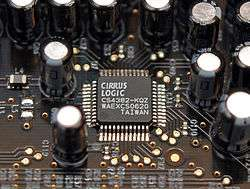8-channel Cirrus Logic CS4382 digital-to-analog converter as used in a sound card.

In electronics, a digital-to-analog converter (DAC, D/A, D–A, D2A, or D-to-A) is a device that converts a digital signal into an analog signal. An analog-to-digital converter (ADC) performs the reverse function.

There are several DAC architectures; the suitability of a DAC for a particular application is determined by three main parameters: resolution, maximum sampling frequency and accuracy. Due to the complexity and the need for precisely matched components, all but the most specialized DACs are implemented as integrated circuits (ICs). Digital-to-analog conversion can degrade a signal, so a DAC should be specified that has insignificant errors in terms of the application.

DACs are commonly used in music players to convert digital data streams into analog audio signals. They are also used in televisions and mobile phones to convert digital video data into analog video signals which connect to the screen drivers to display monochrome or color images. These two applications use DACs at opposite ends of the speed/resolution trade-off. The audio DAC is a low speed high resolution type while the video DAC is a high speed low to medium resolution type. Discrete DACs would typically be extremely high speed low resolution power hungry types, as used in military radar systems. Very high speed test equipment, especially sampling oscilloscopes, may also use discrete DACs.

## Overview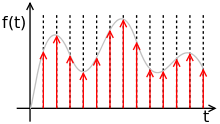Ideally sampled signal.

A DAC converts an abstract finite-precision number (usually a fixed-point binary number) into a physical quantity (e.g., a voltage or a pressure). In particular, DACs are often used to convert finite-precision time series data to a continually varying physical signal.

An ideal DAC converts the abstract numbers into a conceptual sequence of impulses that are then processed by a reconstruction filter using some form of interpolation to fill in data between the impulses. A conventional practical DAC converts the numbers into a piecewise constant function made up of a sequence of rectangular functions that is modeled with the zero-order hold. Other DAC methods such as those based on delta-sigma modulation) produce a pulse-density modulated output that can be similarly filtered to produce a smoothly varying signal.

As per the Nyquist–Shannon sampling theorem, a DAC can reconstruct the original signal from the sampled data provided that its bandwidth meets certain requirements (e.g., a baseband signal with bandwidth less than the Nyquist frequency). Digital sampling introduces quantization error that manifests as low-level noise added to the reconstructed signal.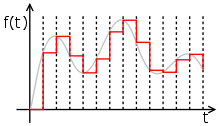Piecewise constant output of a conventional DAC lacking a reconstruction filter. In a practical DAC, a filter or the finite bandwidth of the device smooths out the step response into a continuous curve.

Instead of impulses, a conventional practical DAC updates the analog voltage at uniform sampling intervals, which is then interpolated via a reconstruction filter to continuously varied levels.

These numbers are written to the DAC, typically with a clock signal that causes each number to be latched in sequence, at which time the DAC output voltage changes rapidly from the previous value to the value represented by the currently latched number. The effect of this is that the output voltage is held in time at the current value until the next input number is latched, resulting in a piecewise constant or staircase-shaped output. This is equivalent to a zero-order hold operation and has an effect on the frequency response of the reconstructed signal.

The fact that DACs output a sequence of piecewise constant values (known as zero-order hold in sample data textbooks) or rectangular pulses causes multiple harmonics above the Nyquist frequency. Usually, these are removed with a low pass filter acting as a reconstruction filter in applications that require it.

Other DAC methods (e.g., methods based on delta-sigma modulation) produce a pulse-density modulated signal that can then be filtered in a similar way to produce a smoothly varying signal.

## Applications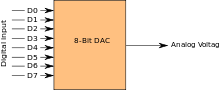A simplified functional diagram of an 8-bit DAC

DACs and ADCs are part of an enabling technology that has contributed greatly to the digital revolution. To illustrate, consider a typical long-distance telephone call. The caller's voice is converted into an analog electrical signal by a microphone, then the analog signal is converted to a digital stream by an ADC. The digital stream is then divided into network packets where it may be sent along with other digital data, not necessarily audio. The packets are then received at the destination, but each packet may take a completely different route and may not even arrive at the destination in the correct time order. The digital voice data is then extracted from the packets and assembled into a digital data stream. A DAC converts this back into an analog electrical signal, which drives an audio amplifier, which in turn drives a loudspeaker, which finally produces sound.

### Audio

Most modern audio signals are stored in digital form (for example MP3s and CDs) and in order to be heard through speakers they must be converted into an analog signal. DACs are therefore found in CD players, digital music players, and PC sound cards.

Specialist standalone DACs can also be found in high-end hi-fi systems. These normally take the digital output of a compatible CD player or dedicated transport (which is basically a CD player with no internal DAC) and convert the signal into an analog line-level output that can then be fed into an amplifier to drive speakers.

Similar digital-to-analog converters can be found in digital speakers such as USB speakers, and in sound cards.

In VoIP (Voice over IP) applications, the source must first be digitized for transmission, so it undergoes conversion via an analog-to-digital converter, and is then reconstructed into analog using a DAC on the receiving party's end.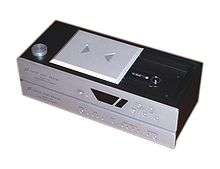### Video

Video sampling tends to work on a completely different scale altogether thanks to the highly nonlinear response both of cathode ray tubes (for which the vast majority of digital video foundation work was targeted) and the human eye, using a "gamma curve" to provide an appearance of evenly distributed brightness steps across the display's full dynamic range - hence the need to use RAMDACs in computer video applications with deep enough colour resolution to make engineering a hardcoded value into the DAC for each output level of each channel impractical (e.g. an Atari ST or Sega Genesis would require 24 such values; a 24-bit video card would need 768...). Given this inherent distortion, it is not unusual for a television or video projector to truthfully claim a linear contrast ratio (difference between darkest and brightest output levels) of 1000:1 or greater, equivalent to 10 bits of audio precision even though it may only accept signals with 8-bit precision and use an LCD panel that only represents 6 or 7 bits per channel.

Video signals from a digital source, such as a computer, must be converted to analog form if they are to be displayed on an analog monitor. As of 2007, analog inputs were more commonly used than digital, but this changed as flat panel displays with DVI and/or HDMI connections became more widespread. A video DAC is, however, incorporated in any digital video player with analog outputs. The DAC is usually integrated with some memory (RAM), which contains conversion tables for gamma correction, contrast and brightness, to make a device called a RAMDAC.

A device that is distantly related to the DAC is the digitally controlled potentiometer, used to control an analog signal digitally.

### Mechanical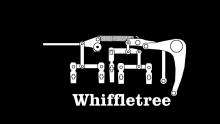IBM Selectric typewriter uses a mechanical digital-to-analog converter to control its typeball.

A one-bit mechanical actuator assumes two positions: one when on, another when off. The motion of several one-bit actuators can be combined and weighted with a whiffletree mechanism to produce finer steps. The IBM Selectric typewriter uses such as system. When a typewriter key is pressed, it moves a metal bar (interposer) down that has several lugs. The lugs are the information bits. When a key is pressed, its interposer is moved by the motor. If a lug is present at one position, it will move the corresponding selector bail (bar); if the lug is not present, the selector bail stays where it is. The discrete motions of the bails are combined by a whiffle tree, and the output controls the rotation and tilt of the Selectric's typeball.

## Types

The most common types of electronic DACs are:

• The pulse-width modulator, the simplest DAC type. A stable current or voltage is switched into a low-pass analog filter with a duration determined by the digital input code. This technique is often used for electric motor speed control, but has many other applications as well.
• Oversampling DACs or interpolating DACs such as the delta-sigma DAC, use a pulse density conversion technique. The oversampling technique allows for the use of a lower resolution DAC internally. A simple 1-bit DAC is often chosen because the oversampled result is inherently linear. The DAC is driven with a pulse-density modulated signal, created with the use of a low-pass filter, step nonlinearity (the actual 1-bit DAC), and negative feedback loop, in a technique called delta-sigma modulation. This results in an effective high-pass filter acting on the quantization (signal processing) noise, thus steering this noise out of the low frequencies of interest into the megahertz frequencies of little interest, which is called noise shaping. The quantization noise at these high frequencies is removed or greatly attenuated by use of an analog low-pass filter at the output (sometimes a simple RC low-pass circuit is sufficient). Most very high resolution DACs (greater than 16 bits) are of this type due to its high linearity and low cost. Higher oversampling rates can relax the specifications of the output low-pass filter and enable further suppression of quantization noise. Speeds of greater than 100 thousand samples per second (for example, 192 kHz) and resolutions of 24 bits are attainable with delta-sigma DACs. A short comparison with pulse-width modulation shows that a 1-bit DAC with a simple first-order integrator would have to run at 3 THz (which is physically unrealizable) to achieve 24 meaningful bits of resolution, requiring a higher-order low-pass filter in the noise-shaping loop. A single integrator is a low-pass filter with a frequency response inversely proportional to frequency and using one such integrator in the noise-shaping loop is a first order delta-sigma modulator. Multiple higher order topologies (such as MASH) are used to achieve higher degrees of noise-shaping with a stable topology.
• The binary-weighted DAC, which contains individual electrical components for each bit of the DAC connected to a summing point. These precise voltages or currents sum to the correct output value. This is one of the fastest conversion methods but suffers from poor accuracy because of the high precision required for each individual voltage or current. Such high-precision components are expensive, so this type of converter is usually limited to 8-bit resolution or less.
• Switched resistor DAC contains a parallel resistor network. Individual resistors are enabled or bypassed in the network based on the digital input.
• Switched current source DAC, from which different current sources are selected based on the digital input.
• Switched capacitor DAC contains a parallel capacitor network. Individual capacitors are connected or disconnected with switches based on the input.
• The R-2R ladder DAC which is a binary-weighted DAC that uses a repeating cascaded structure of resistor values R and 2R. This improves the precision due to the relative ease of producing equal valued-matched resistors (or current sources).
• The Successive-Approximation or Cyclic DAC, which successively constructs the output during each cycle. Individual bits of the digital input are processed each cycle until the entire input is accounted for.
• The thermometer-coded DAC, which contains an equal resistor or current-source segment for each possible value of DAC output. An 8-bit thermometer DAC would have 255 segments, and a 16-bit thermometer DAC would have 65,535 segments. This is perhaps the fastest and highest precision DAC architecture but at the expense of high cost. Conversion speeds of >1 billion samples per second have been reached with this type of DAC.
• Hybrid DACs, which use a combination of the above techniques in a single converter. Most DAC integrated circuits are of this type due to the difficulty of getting low cost, high speed and high precision in one device.
• The segmented DAC, which combines the thermometer-coded principle for the most significant bits and the binary-weighted principle for the least significant bits. In this way, a compromise is obtained between precision (by the use of the thermometer-coded principle) and number of resistors or current sources (by the use of the binary-weighted principle). The full binary-weighted design means 0% segmentation, the full thermometer-coded design means 100% segmentation.
• Most DACs, shown earlier in this list, rely on a constant reference voltage to create their output value. Alternatively, a multiplying DAC takes a variable input voltage for their conversion. This puts additional design constraints on the bandwidth of the conversion circuit.

## Performance

DACs are very important to system performance. The most important characteristics of these devices are:

Resolution
The number of possible output levels the DAC is designed to reproduce. This is usually stated as the number of bits it uses, which is the base two logarithm of the number of levels. For instance a 1 bit DAC is designed to reproduce 2 (21) levels while an 8 bit DAC is designed for 256 (28) levels. Resolution is related to the effective number of bits which is a measurement of the actual resolution attained by the DAC. Resolution determines color depth in video applications and audio bit depth in audio applications.
Maximum sampling rate
A measurement of the maximum speed at which the DACs circuitry can operate and still produce the correct output. As stated above, the Nyquist–Shannon sampling theorem defines a relationship between this and the bandwidth of the sampled signal.
Monotonicity
The ability of a DAC's analog output to move only in the direction that the digital input moves (i.e., if the input increases, the output doesn't dip before asserting the correct output.) This characteristic is very important for DACs used as a low frequency signal source or as a digitally programmable trim element.
Total harmonic distortion and noise (THD+N)
A measurement of the distortion and noise introduced to the signal by the DAC. It is expressed as a percentage of the total power of unwanted harmonic distortion and noise that accompany the desired signal. This is a very important DAC characteristic for dynamic and small signal DAC applications.
Dynamic range
A measurement of the difference between the largest and smallest signals the DAC can reproduce expressed in decibels. This is usually related to resolution and noise floor.

Other measurements, such as phase distortion and jitter, can also be very important for some applications, some of which (e.g. wireless data transmission, composite video) may even rely on accurate production of phase-adjusted signals.

Linear PCM audio sampling usually works on the basis of each bit of resolution being equivalent to 6 decibels of amplitude (a 2x increase in volume or precision).

Non-linear PCM encodings (A-law / μ-law, ADPCM, NICAM) attempt to improve their effective dynamic ranges by a variety of methods - logarithmic step sizes between the output signal strengths represented by each data bit (trading greater quantisation distortion of loud signals for better performance of quiet signals)

## Figures of merit

• Static performance:
• Differential nonlinearity (DNL) shows how much two adjacent code analog values deviate from the ideal 1 LSB step.
• Integral nonlinearity (INL) shows how much the DAC transfer characteristic deviates from an ideal one. That is, the ideal characteristic is usually a straight line; INL shows how much the actual voltage at a given code value differs from that line, in LSBs (1 LSB steps).
• Gain
• Offset
• Noise is ultimately limited by the thermal noise generated by passive components such as resistors. For audio applications and in room temperatures, such noise is usually a little less than 1 μV (microvolt) of white noise. This limits performance to less than 20~21 bits even in 24-bit DACs.
• Frequency domain performance
• Spurious-free dynamic range (SFDR) indicates in dB the ratio between the powers of the converted main signal and the greatest undesired spur.
• Signal-to-noise and distortion ratio (SNDR) indicates in dB the ratio between the powers of the converted main signal and the sum of the noise and the generated harmonic spurs
• i-th harmonic distortion (HDi) indicates the power of the i-th harmonic of the converted main signal
• Total harmonic distortion (THD) is the sum of the powers of all HDi
• If the maximum DNL error is less than 1 LSB, then the D/A converter is guaranteed to be monotonic. However, many monotonic converters may have a maximum DNL greater than 1 LSB.
• Time domain performance:
• Glitch impulse area (glitch energy)
• Response uncertainty
• Time nonlinearity (TNL)